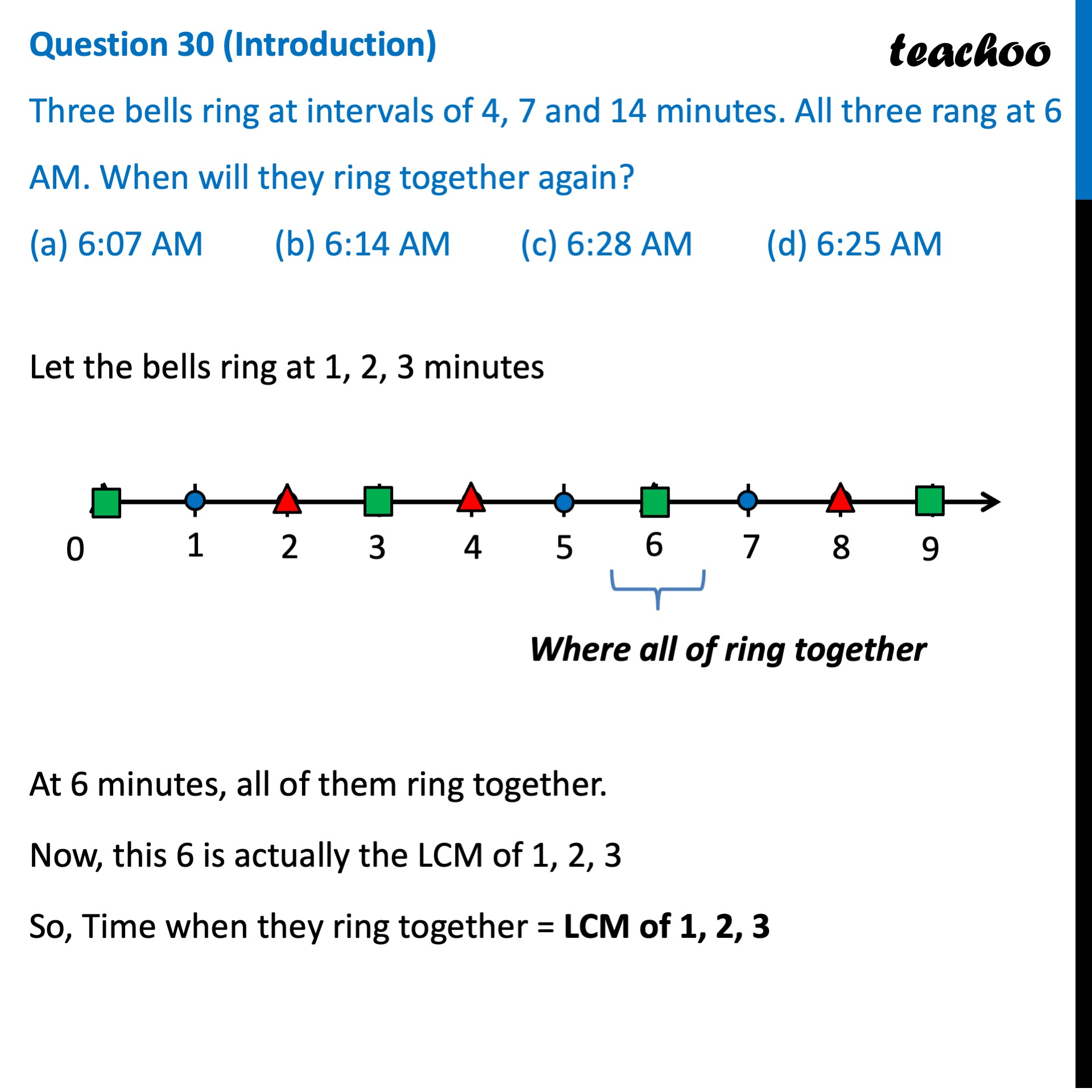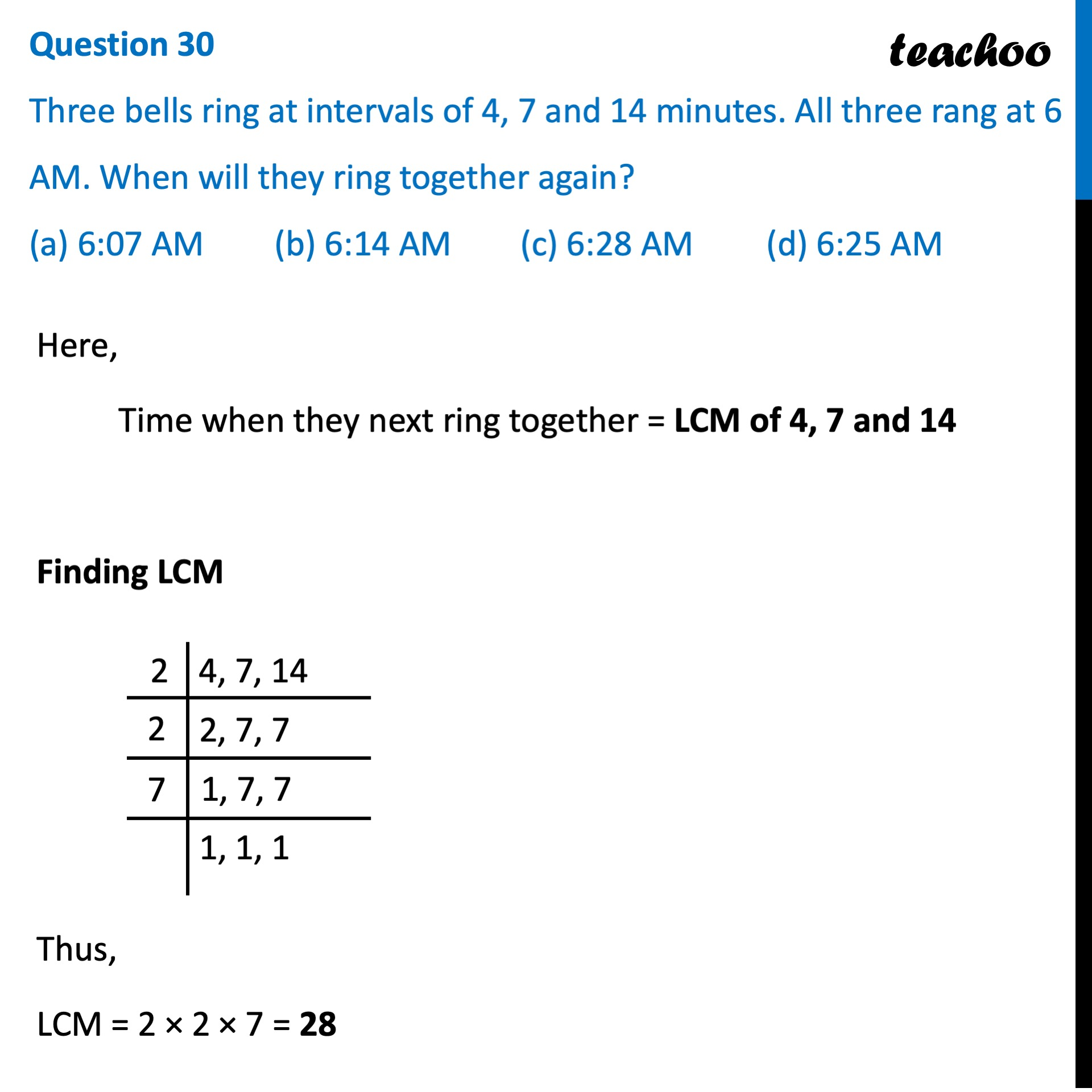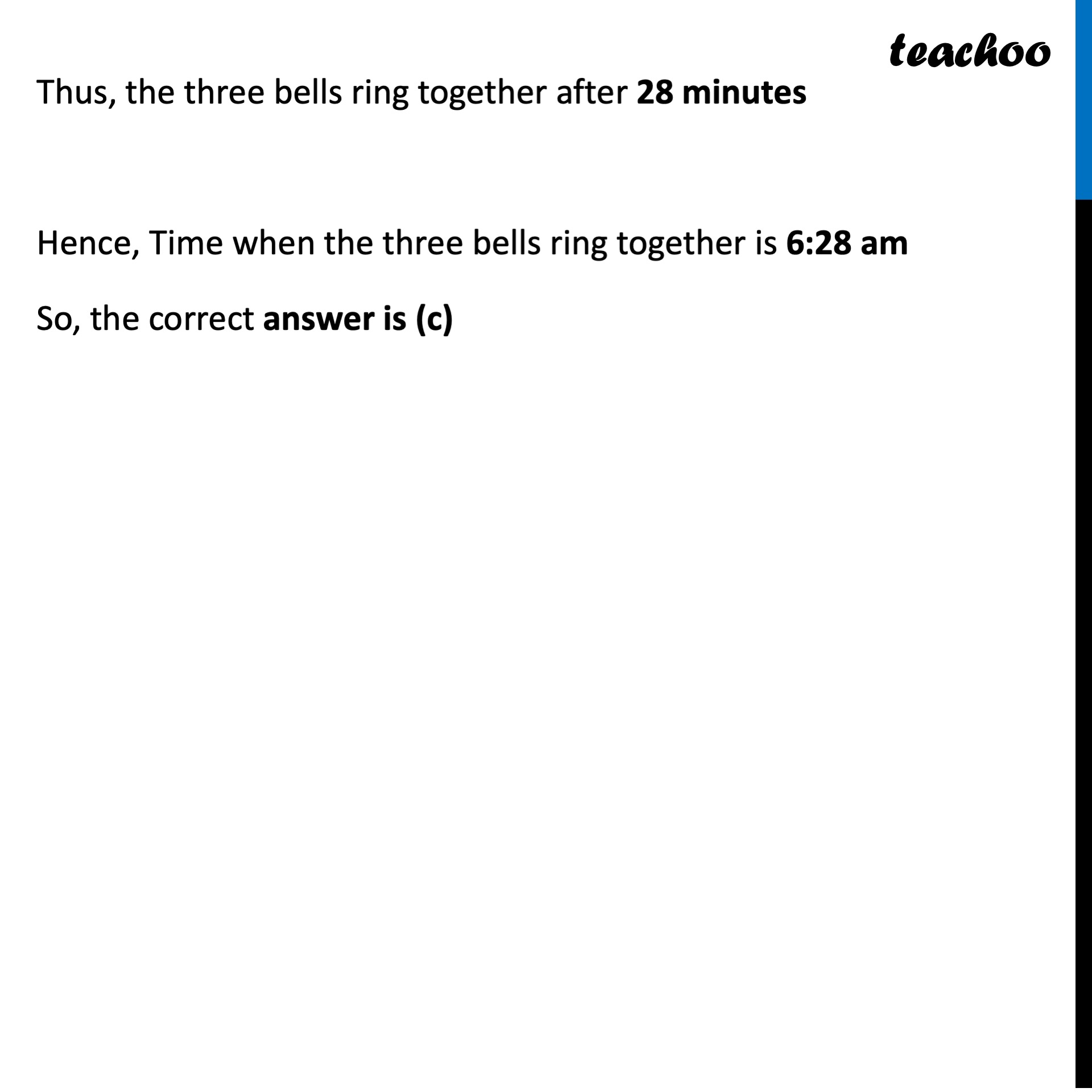## (a) 6:07 AM   (b) 6:14 AM   (c) 6:28 AM   (d) 6:25 AM

This question is inspired from Question 21 - CBSE Sample Papers (2021 Boards) - Maths Standard1. Class 10
2. Solutions of Sample Papers for Class 10 Boards
3. CBSE Class 10 Sample Paper for 2022 Boards - Maths Basic [MCQ]

Transcript

Question 30 Three bells ring at intervals of 4, 7 and 14 minutes. All three rang at 6 AM. When will they ring together again? (a) 6:07 AM (b) 6:14 AM (c) 6:28 AM (d) 6:25 AM Let the bells ring at 1, 2, 3 minutes At 6 minutes, all of them ring together. Now, this 6 is actually the LCM of 1, 2, 3 So, Time when they ring together = LCM of 1, 2, 3 Here, Time when they next ring together = LCM of 4, 7 and 14 Finding LCM Thus, LCM = 2 × 2 × 7 = 28 Thus, the three bells ring together after 28 minutes Hence, Time when the three bells ring together is 6:28 am So, the correct answer is (c)